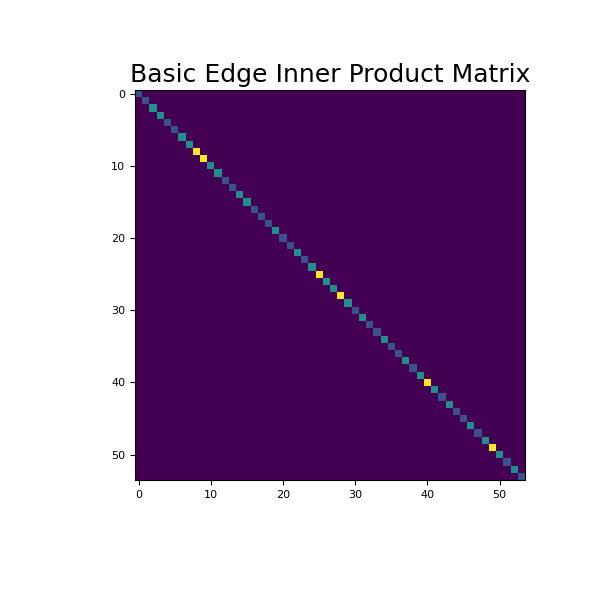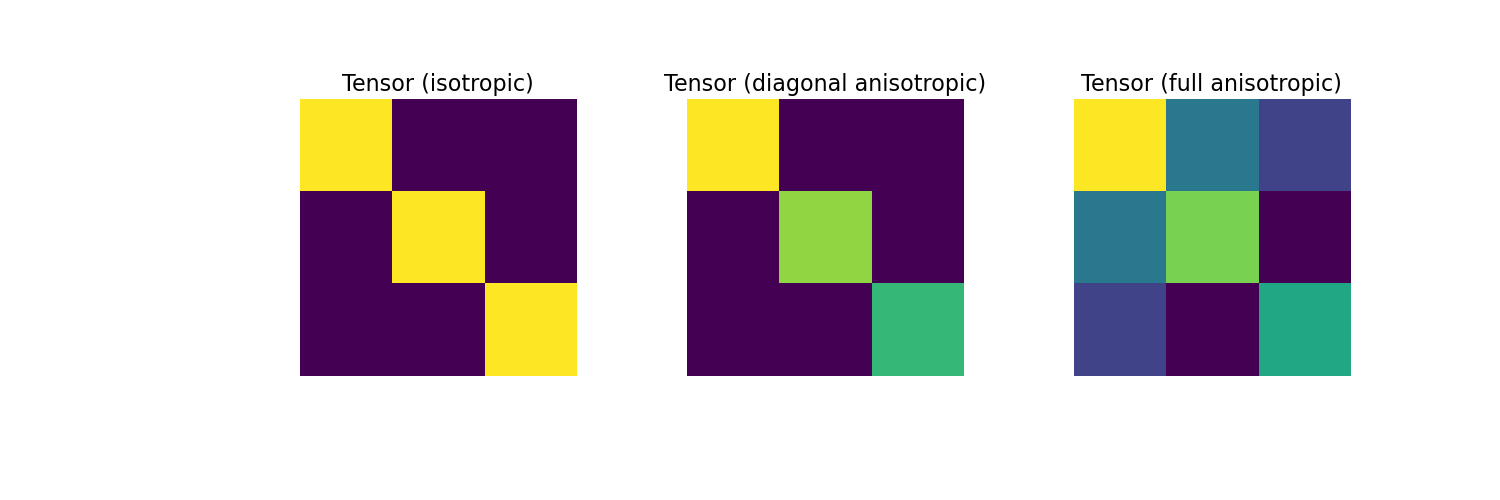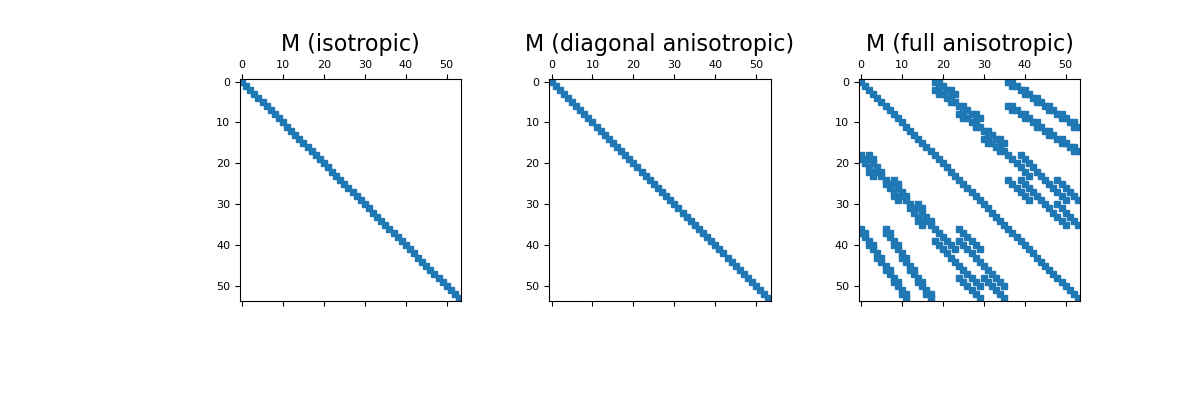# discretize.TreeMesh.get_edge_inner_product¶

TreeMesh.get_edge_inner_product(model=None, invert_model=False, invert_matrix=False, do_fast=True, **kwargs)[source]

Generate the edge inner product matrix or its inverse.

This method generates the inner product matrix (or its inverse) when discrete variables are defined on mesh edges. It is also capable of constructing the inner product matrix when physical properties are defined in the form of constitutive relations. For a comprehensive description of the inner product matrices that can be constructed with get_edge_inner_product, see Notes.

Parameters
model

Parameters defining the material properties for every cell in the mesh. Inner product matrices can be constructed for the following cases:

• None : returns the basic inner product matrix

• (n_cells) numpy.ndarray : returns inner product matrix for an isotropic model. The array contains a scalar physical property value for each cell.

• (n_cells, dim) numpy.ndarray : returns inner product matrix for diagonal anisotropic case. Columns are ordered np.c_[σ_xx, σ_yy, σ_zz]. This can also a be a 1D array with the same number of total elements in column major order.

• (n_cells, 3) numpy.ndarray (dim is 2) or (n_cells, 6) numpy.ndarray (dim is 3) : returns inner product matrix for full tensor properties case. Columns are ordered np.c_[σ_xx, σ_yy, σ_zz, σ_xy, σ_xz, σ_yz] This can also be a 1D array with the same number of total elements in column major order.

invert_modelbool, optional

The inverse of model is used as the physical property.

invert_matrixbool, optional

Teturns the inverse of the inner product matrix. The inverse not implemented for full tensor properties.

do_fastbool, optional

Do a faster implementation (if available).

Returns
(n_edges, n_edges) scipy.sparse.csr_matrix

inner product matrix

Notes

For continuous vector quantities $$\vec{u}$$ and $$\vec{w}$$ whose discrete representations $$\mathbf{u}$$ and $$\mathbf{w}$$ live on the edges, get_edge_inner_product constructs the inner product matrix $$\mathbf{M_\ast}$$ (or its inverse $$\mathbf{M_\ast^{-1}}$$) for the following cases:

Basic Inner Product: the inner product between $$\vec{u}$$ and $$\vec{w}$$.

$\langle \vec{u}, \vec{w} \rangle = \mathbf{u^T \, M \, w}$

Isotropic Case: the inner product between $$\vec{u}$$ and $$\sigma \vec{w}$$ where $$\sigma$$ is a scalar function.

$\langle \vec{u}, \sigma \vec{w} \rangle = \mathbf{u^T \, M_\sigma \, w}$

Tensor Case: the inner product between $$\vec{u}$$ and $$\Sigma \vec{w}$$ where $$\Sigma$$ is tensor function; $$\sigma_{xy} = \sigma_{xz} = \sigma_{yz} = 0$$ for diagonal anisotropy.

$\begin{split}\langle \vec{u}, \Sigma \vec{w} \rangle = \mathbf{u^T \, M_\Sigma \, w} \;\;\; \textrm{where} \;\;\; \Sigma = \begin{bmatrix} \sigma_{xx} & \sigma_{xy} & \sigma_{xz} \\ \sigma_{xy} & \sigma_{yy} & \sigma_{yz} \\ \sigma_{xz} & \sigma_{yz} & \sigma_{zz} \end{bmatrix}\end{split}$

Examples

Here we provide some examples of edge inner product matrices. For simplicity, we will work on a 2 x 2 x 2 tensor mesh. As seen below, we begin by constructing and imaging the basic edge inner product matrix.

>>> from discretize import TensorMesh
>>> import matplotlib.pyplot as plt
>>> import numpy as np
>>> import matplotlib as mpl

>>> h = np.ones(2)
>>> mesh = TensorMesh([h, h, h])
>>> Me = mesh.get_edge_inner_product()

>>> fig = plt.figure(figsize=(6, 6))
>>> ax.imshow(Me.todense())
>>> ax.set_title('Basic Edge Inner Product Matrix', fontsize=18)
>>> plt.show()Next, we consider the case where the physical properties of the cells are defined by consistutive relations. For the isotropic, diagonal anisotropic and full tensor cases, we show the physical property tensor for a single cell.

Define 4 constitutive parameters and define the tensor for each cell for isotropic, diagonal and tensor cases.

>>> sig1, sig2, sig3, sig4, sig5, sig6 = 6, 5, 4, 3, 2, 1
>>> sig_iso_tensor = sig1 * np.eye(3)
>>> sig_diag_tensor = np.diag(np.array([sig1, sig2, sig3]))
>>> sig_full_tensor = np.array([
...     [sig1, sig4, sig5],
...     [sig4, sig2, sig6],
...     [sig5, sig6, sig3]
... ])


Then plot the matrix entries,

Expand to show scripting for plot
>>> fig = plt.figure(figsize=(15, 5))
>>> ax1.imshow(sig_iso_tensor)
>>> ax1.axis('off')
>>> ax1.set_title("Tensor (isotropic)", fontsize=16)
>>> ax2.imshow(sig_diag_tensor)
>>> ax2.axis('off')
>>> ax2.set_title("Tensor (diagonal anisotropic)", fontsize=16)
>>> ax3.imshow(sig_full_tensor)
>>> ax3.axis('off')
>>> ax3.set_title("Tensor (full anisotropic)", fontsize=16)
>>> plt.show()


(png, pdf)Here construct and image the edge inner product matrices for the isotropic, diagonal anisotropic and full tensor cases. Spy plots are used to demonstrate the sparsity of the inner product matrices.

Isotropic case:

>>> v = np.ones(mesh.nC)
>>> sig = sig1 * v
>>> M1 = mesh.get_edge_inner_product(sig)


Diagonal anisotropic case:

>>> sig = np.c_[sig1*v, sig2*v, sig3*v]
>>> M2 = mesh.get_edge_inner_product(sig)


Full anisotropic

>>> sig = np.tile(np.c_[sig1, sig2, sig3, sig4, sig5, sig6], (mesh.nC, 1))
>>> M3 = mesh.get_edge_inner_product(sig)


Then plot the sparse representation,

Expand to show scripting for plot
>>> fig = plt.figure(figsize=(12, 4))
>>> ax1.spy(M1, ms=5)
>>> ax1.set_title("M (isotropic)", fontsize=16)